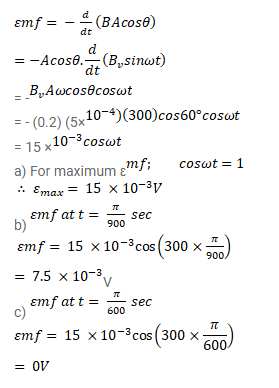# A conducting loop of area

Question:

A conducting loop of area $5.0 \mathrm{~cm}^{2}$ is placed in a magnetic field which varies sinusoidally with time as $B=B_{v}$ sin $\omega t$ where $B_{v}=0.20 \mathrm{~T}$ and $\omega=300 \mathrm{~s}^{-1}$. The normal to the coil makes an angle of $60^{\circ}$ with the field. Find (a) The maximum emf induced in the coil, (b) the emf induced at $r=(\pi / 900) s$ and (c) the emf induced at $t=\left(\frac{\pi}{600}\right) s$

Solution: